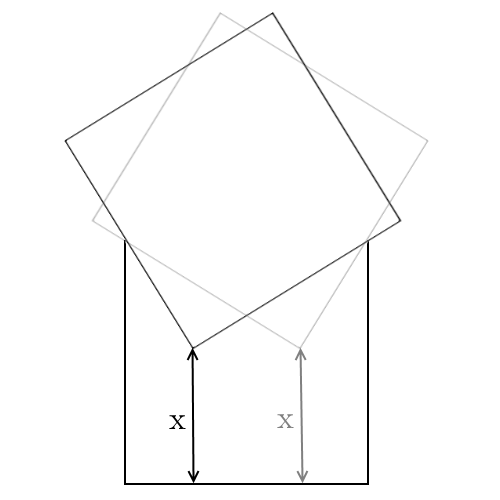# Boxed In

Geometry Level 3A square with side $1$ falls on top of an open top square box with side $1$ (pictured above). Assuming the square doesn't fall completely in the box, find the smallest value for $x$, where $x$ is the the line that connects the vertex of the square inside the box to the box base perpendicularly.

×